# 4. 文本建模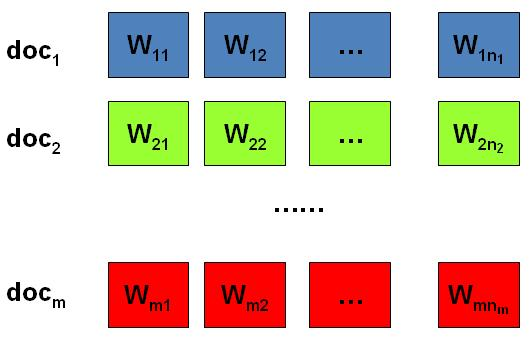包含$M$篇文档的语料库

• 上帝都有什么样的骰子；
• 上帝是如何抛掷这些骰子的；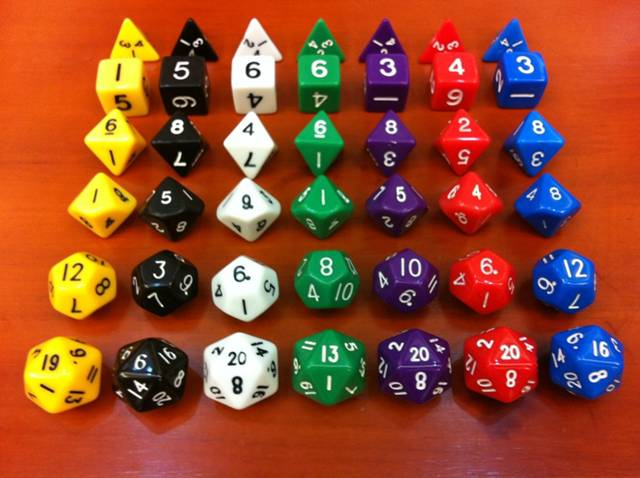## 4.1 Unigram Model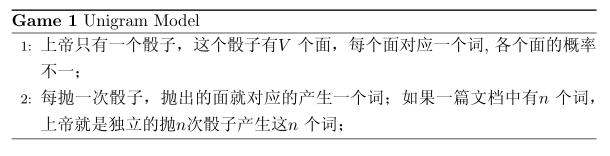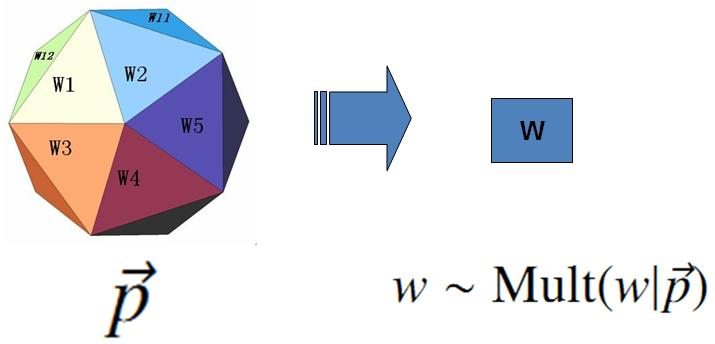$$p(\overrightarrow{w}) = p(w_1, w_2, \cdots, w_n) = p(w_1)p(w_2) \cdots p(w_n)$$

$$p(\mathcal{W})= p(\overrightarrow{w_1})p(\overrightarrow{w_2})\cdots p(\overrightarrow{w_m})$$

$$p(\overrightarrow{n}) = Mult(\overrightarrow{n}|\overrightarrow{p}, N) = \binom{N}{\overrightarrow{n}} \prod_{k=1}^V p_k^{n_k}$$

\begin{align*} p(\mathcal{W})= p(\overrightarrow{w_1})p(\overrightarrow{w_2}) \cdots p(\overrightarrow{w_m}) = \prod_{k=1}^V p_k^{n_k} \end{align*}

$$\hat{p_i} = \frac{n_i}{N} .$$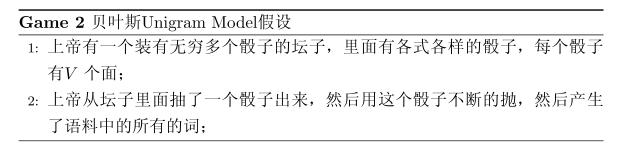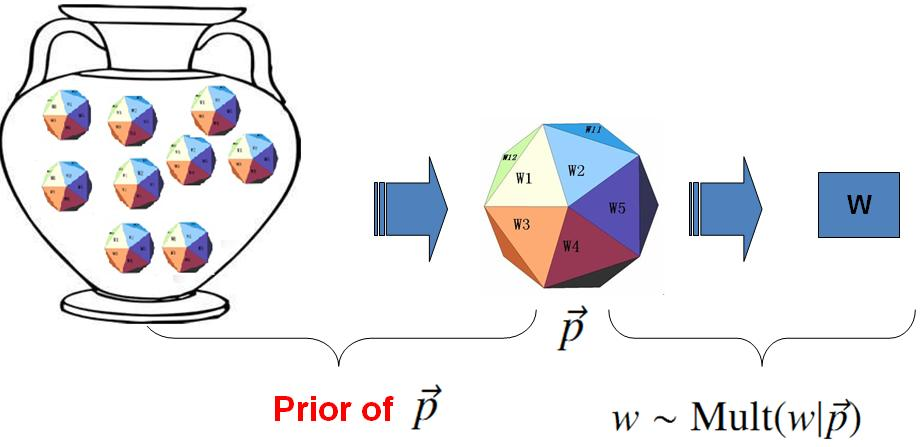$$p(\mathcal{W}) = \int p(\mathcal{W}|\overrightarrow{p}) p(\overrightarrow{p})d\overrightarrow{p}$$

$$p(\overrightarrow{n}) = Mult(\overrightarrow{n}|\overrightarrow{p}, N)$$

$$Dir(\overrightarrow{p}|\overrightarrow{\alpha})= \frac{1}{\Delta(\overrightarrow{\alpha})} \prod_{k=1}^V p_k^{\alpha_k -1}，\quad \overrightarrow{\alpha}=(\alpha_1, \cdots, \alpha_V)$$

$$\Delta(\overrightarrow{\alpha}) = \int \prod_{k=1}^V p_k^{\alpha_k -1} d\overrightarrow{p} .$$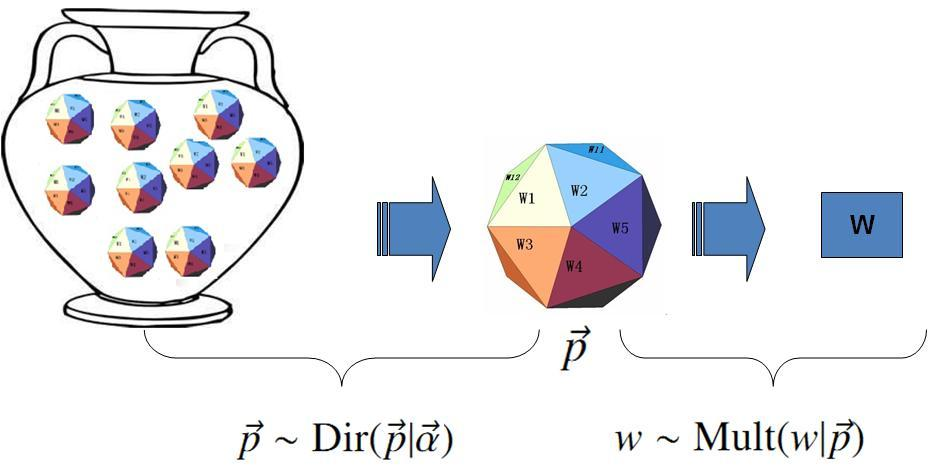Dirichlet 先验下的 Unigram Model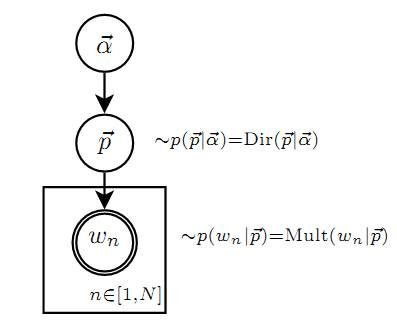Unigram Model的概率图模型

Dirichlet 先验 + 多项分布的数据$\rightarrow$后验分布为 Dirichlet 分布

$$Dir(\overrightarrow{p}|\overrightarrow{\alpha}) + MultCount(\overrightarrow{n})= Dir(\overrightarrow{p}|\overrightarrow{\alpha}+\overrightarrow{n})$$

\begin{equation} p(\overrightarrow{p}|\mathcal{W},\overrightarrow{\alpha}) = Dir(\overrightarrow{p}|\overrightarrow{n}+ \overrightarrow{\alpha}) = \frac{1}{\Delta(\overrightarrow{n}+\overrightarrow{\alpha})} \prod_{k=1}^V p_k^{n_k + \alpha_k -1} d\overrightarrow{p} \end{equation}

$$E(\overrightarrow{p}) = \Bigl(\frac{n_1 + \alpha_1}{\sum_{i=1}^V(n_i + \alpha_i)}, \frac{n_2 + \alpha_2}{\sum_{i=1}^V(n_i + \alpha_i)}, \cdots, \frac{n_V + \alpha_V}{\sum_{i=1}^V(n_i + \alpha_i)} \Bigr)$$

\begin{equation} \label{dirichlet-parameter-estimation} \hat{p_i} = \frac{n_i + \alpha_i}{\sum_{i=1}^V(n_i + \alpha_i)} \end{equation}

\begin{align} p(\mathcal{W}|\overrightarrow{\alpha}) & = \int p(\mathcal{W}|\overrightarrow{p}) p(\overrightarrow{p}|\overrightarrow{\alpha})d\overrightarrow{p} \notag \\ & = \int \prod_{k=1}^V p_k^{n_k} Dir(\overrightarrow{p}|\overrightarrow{\alpha}) d\overrightarrow{p} \notag \\ & = \int \prod_{k=1}^V p_k^{n_k} \frac{1}{\Delta(\overrightarrow{\alpha})} \prod_{k=1}^V p_k^{\alpha_k -1} d\overrightarrow{p} \notag \\ & = \frac{1}{\Delta(\overrightarrow{\alpha})} \int \prod_{k=1}^V p_k^{n_k + \alpha_k -1} d\overrightarrow{p} \notag \\ & = \frac{\Delta(\overrightarrow{n}+\overrightarrow{\alpha})}{\Delta(\overrightarrow{\alpha})} \label{likelihood-dir-mult} \end{align}

## 4.2 Topic Model 和 PLSA

• 说到语言学，我们容易想到的词包括：语法、句子、乔姆斯基、句法分析、主语…；
• 谈论概率统计，我们容易想到以下一些词: 概率、模型、均值、方差、证明、独立、马尔科夫链、…；
• 谈论计算机，我们容易想到的词是： 内存、硬盘、编程、二进制、对象、算法、复杂度…；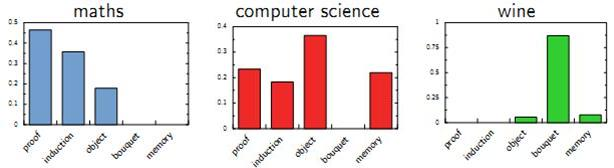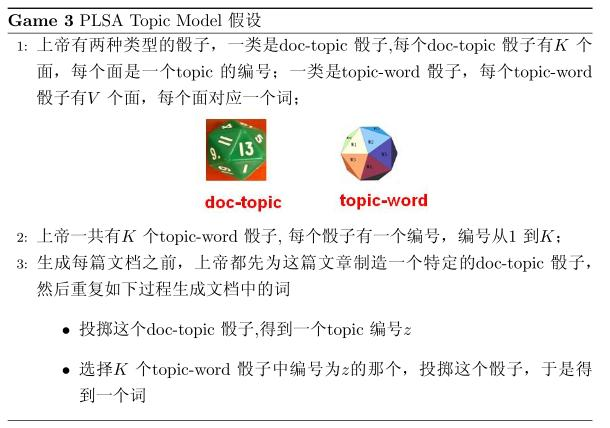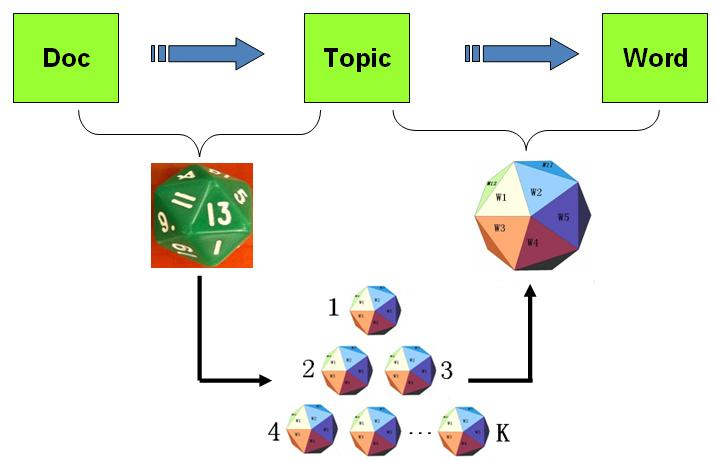PLSA模型的文档生成过程

$$p(w|d_m) = \sum_{z=1}^K p(w|z)p(z|d_m) = \sum_{z=1}^K \varphi_{zw} \theta_{mz}$$

$$p(\overrightarrow{w}|d_m) = \prod_{i=1}^n \sum_{z=1}^K p(w_i|z)p(z|d_m) = \prod_{i=1}^n \sum_{z=1}^K \varphi_{zw_i} \theta_{dz}$$